Students can download Maths Chapter 4 Geometry Ex 4.3 Questions and Answers, Notes, Samacheer Kalvi 10th Maths Guide Pdf helps you to revise the complete Tamilnadu State Board New Syllabus, helps students complete homework assignments and to score high marks in board exams.

## Tamilnadu Samacheer Kalvi 10th Maths Solutions Chapter 4 Geometry Ex 4.3

Question 1.
A man goes 18 m due east and then 24 m due north. Find the distance of his current position from the starting point?
Solution:
Let the initial position of the man be “O” and his final
position be “B”.
By Pythagoras theorem
In the right ∆ OAB,OB2 = OA2 + AB2
= 182 + 242
= 324 + 576 = 900
OB = $$\sqrt { 900 }$$ = 30
The distance of his current position is 30 mQuestion 2.
There are two paths that one can choose to go from Sarah’s house to James house. One way is to take C street, and the other way requires to take A street and then B street. How much shorter is the direct path along C street? (Using figure).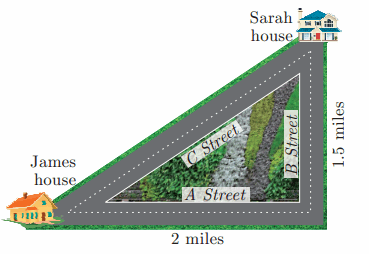Solution:
Distance between Sarah House and James House using “C street”.
AC2 = AB2 + BC2
= 22 + 1.52
= 4 + 2.25 = 6.25
AC = $$\sqrt { 6.25 }$$
AC = 2.5 milesDistance covered by using “A Street” and “B Street”
= (2 + 1.5) miles = 3.5 miles
Difference in distance = 3.5 miles – 2.5 miles = 1 mileQuestion 3.
To get from point A to point B you must avoid walking through a pond. You must walk 34 m south and 41 m east. To the nearest meter, how many meters would be saved if it were possible to make a way through the pond?
Solution:
In the right ∆ABC,
By Pythagoras theorem
AC2= AB2 + BC2 = 342 + 412
= 1156 + 1681 = 2837
AC = $$\sqrt { 2837 }$$
= 53.26 m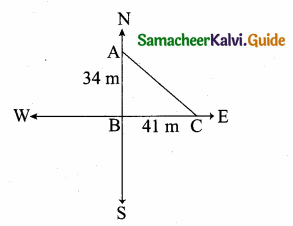Through A one must walk (34m + 41m) 75 m to reach C.
The difference in Distance = 75 – 53.26
= 21.74 mQuestion 4.
In the rectangle WXYZ, XY + YZ = 17 cm, and XZ + YW = 26 cm.
Calculate the length and breadth of the rectangle?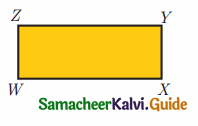Solution:
Let the length of the rectangle be “a” and the breadth of the rectangle be “b”.
XY + YZ = 17 cm
b + a = 17 …….. (1)
In the right ∆ WXZ,
XZ2 = WX2 + WZ2
(XZ)2 = a2 + b2XZ = $$\sqrt{a^{2}+b^{2}}$$
Similarly WY = $$\sqrt{a^{2}+b^{2}}$$ ⇒ XZ + WY = 26
2 $$\sqrt{a^{2}+b^{2}}$$ = 26 ⇒ $$\sqrt{a^{2}+b^{2}}$$ = 13
Squaring on both sides
a2 + b2 = 169
(a + b)2 – 2ab = 169
172 – 2ab = 169 ⇒ 289 – 169 = 2 ab
120 = 2 ab ⇒ ∴ ab = 60
a = $$\frac { 60 }{ b }$$ ….. (2)
Substituting the value of a = $$\frac { 60 }{ b }$$ in (1)
$$\frac { 60 }{ b }$$ + b = 17
b2 – 17b + 60 = 0
(b – 2) (b – 5) = 0
b = 12 or b = 5If b = 12 ⇒ a = 5
If b = 6 ⇒ a = 12
Lenght = 12 m and breadth = 5 mQuestion 5.
The hypotenuse of a right triangle is 6 m more than twice of the shortest side. If the third side is 2 m less than the hypotenuse, find the sides of the triangle.
Solution:
Let the shortest side of the right ∆ be x.
∴ Hypotenuse = 6 + 2x
Third side = 2x + 6 – 2
= 2x + 4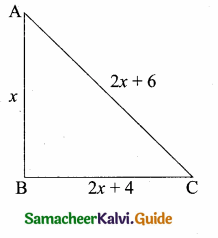In the right triangle ABC,
AC2 = AB2 + BC2
(2x + 6)2 = x2 + (2x + 4)2
4x2 + 36 + 24x = x2 + 4x2 + 16 + 16x
0 = x2 – 24x + 16x – 36 + 16
∴ x2 – 8x – 20 = 0
(x – 10) (x + 2) = 0
x – 10 = 0 or x + 2 = 0x = 10 or x = -2 (Negative value will be omitted)
The side AB = 10 m
The side BC = 2 (10) + 4 = 24 m
Hypotenuse AC = 2(10) + 6 = 26 mQuestion 6.
5 m long ladder is placed leaning towards a vertical wall such that it reaches the wall at a point 4m high. If the foot of the ladder is moved 1.6 m towards the wall, then find the distance by which the top of the ladder would slide upwards on the wall.
Solution:
“C” is the position of the foot of the ladder “A” is the position of the top of the ladder.
In the right ∆ABC,
BC2 = AC2 – AB2 = 52 – 42
= 25 – 16 = 9
BC = $$\sqrt { 9 }$$ = 3m.
When the foot of the ladder moved 1.6 m toward the wall.
The distance between the foot of the ladder to the ground is
BE = 3 – 1.6 m
= 1.4 m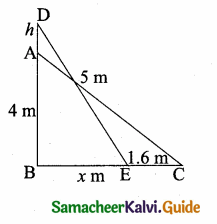Let the distance moved upward on the wall be “h” m
The ladder touch the wall at (4 + h) M
In the right triangle BED,
ED2 = AB2 + BE2
52 = (4 + h)2 + (1.4)2
25 – 1.96= (4 + h)2
∴ 4 + h = $$\sqrt { 23.04 }$$
4 + h = 4. 8 m
h = 4.8 – 4
= 0.8 m
Distance moved upward on the wall = 0.8 mQuestion 7.
The perpendicular PS on the base QR of a ∆PQR intersects QR at S, such that QS = 3 SR. Prove that 2PQ2 = 2PR2 + QR2.
Solution:
Given QS = 3SR
QR = QS + SR
= 3SR + SR = 4SR
SR = $$\frac { 1 }{ 4 }$$ QR …..(1)
QS = 3SR
SR = $$\frac { QS }{ 3 }$$ ……..(2)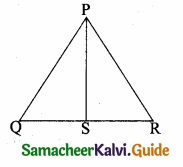From (1) and (2) we get
$$\frac { 1 }{ 4 }$$ QR = $$\frac { QS }{ 3 }$$
∴ QS = $$\frac { 3 }{ 4 }$$ QR ………(3)
In the right ∆ PQS,
PQ2 = PS2 + QS2 ……….(4)
Similarly in ∆ PSR
PR2 = PS2 + SR2 ………..(5)
Subtract (4) and (5)
PQ2 – PR2 = PS2 + QS2 – PS2 – SR2
= QS2 – SR2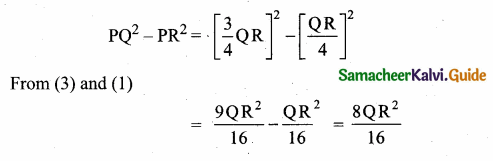PQ2 – PR2 = $$\frac { 1 }{ 2 }$$ QR2
2PQ2 – 2PR2 = QR2
2PQ2 = 2PR2 + QR2
Hence the proved.Question 8.
In the adjacent figure, ABC is a right angled triangle with right angle at B and points D, E trisect BC. Prove that 8AE2 = 3AC2 + 5AD2.
Solution:
Since the Points D, E trisect BC.
BD = DE = CE
Let BD = DE = CE = x
BE = 2x and BC = 3xIn the right ∆ABD,
AD2 = AB2 + x2 ……….(1)
In the right ∆ABE,
AE2 = AB2 + 2BE2
AE2 = AB2 + 4X2 ………..(2) (BE = 2x)
In the right ∆ABC
AC2 = AB2 + BC2
AC2 = AB2 + 9x2 …………… (3) (BC = 3x)